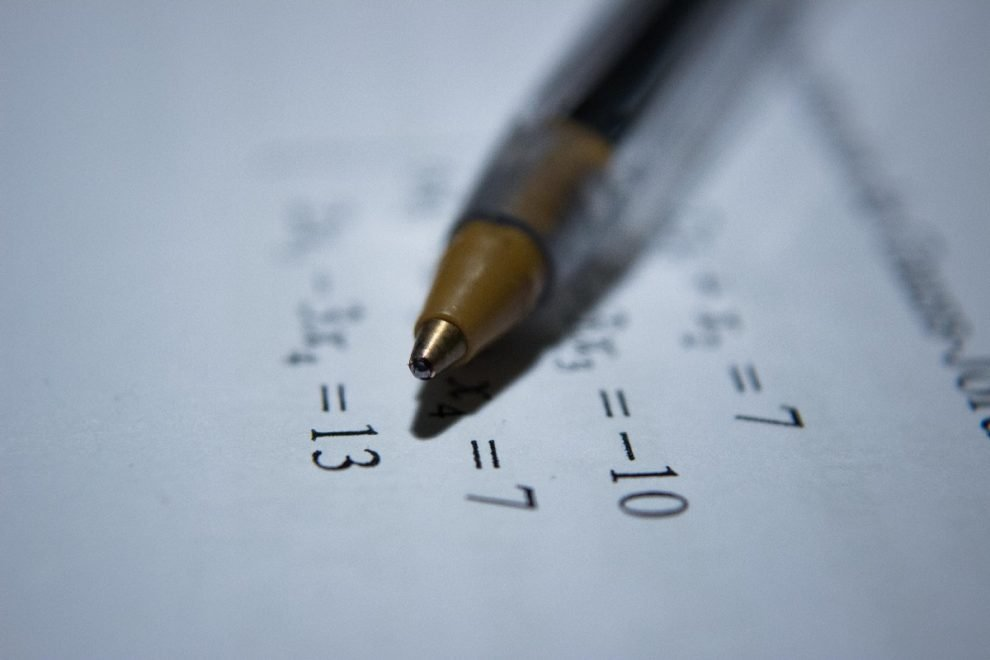Education

# Bayes Theorem### Introduction to Bayes Theorem

In Probability, the theorem that helps in determining the probability of any event that has already taken place is known as the Bayes Theorem. The theorem is named after Reverend Thomas Bayes, a British mathematician. Bayes theorem has many usages and applications, for example, it can be applied in determining the accuracy of medical test results, or in finance with the intention of risk evaluation. Bayes theorem is a mathematical formula to determine conditional probability.

### Introduction to Probability

Probability depicts the possibility of the occurrence of any event. In our daily lives, we often come across certain situations where we are required to predict the possibility of the occurrence of an event. We then say that there might be a probability of the event occurring or not. Some examples of applications of probability are in games, a business where predictions are made based on probability, etc.

The Probability of an outcome is calculated by dividing the favorable number of outcomes by the total number of possible outcomes. The value of probability lies between 0 and 1 since favorable outcomes can never be greater than the possible outcomes.

### What is Probability?

Probability can be defined as the ratio of the number of favorable outcomes and the total number of possible outcomes. The formula with which probability is expressed:

Probability of the event=x/n; where x=the favorable outcomes and n=the total number of possible outcomes.

### Types of Probability

There are 3 different kinds of probability based on the approach followed to get the outcome:

• Classical Probability- Classical probability is used to determine the probability considering the given set of possible outcomes by an event and each given outcome is equally likely to happen.
• Empirical Probability- This is the method that is used to determine the probability of an event through experiments performed.
• Subjective Probability- This one involves an individual’s own belief in the likeliness of an event occurring.
• Axiomatic Probability- In axiomatic probability, a set of rules made by Kolmogorov are applied to all types of probability including frequentist and classical probability.

### What is Bayes Theorem?

Bayes Theorem is also referred to as the Bayes Rule or Bayes Law. It is a theorem that is used to calculate the probability of an event considering the occurrence of prior or existing events. This theorem is applied to find out conditional probability. The expression of the Bayes Theorem:

P(A|B) = P(B|A) P(A)P(B)

Conditions are-

P(A)= likeliness of occurrence of event A, P(B)=likeliness of occurrence of event B, P(A/B)= possibility of occurrence of A when B has already taken place, and P(B/A)=possibility of occurrence of B while A has already happened.

### Some Important Facts to Remember about Bayes Theorem

• Conditional Probability is determined with the application of the Bayes Theorem.
• If two events such as A and B are independent then, P(A|B) =P(A), and P(B|A) =P(B).
• The Bayes Theorem is also used to calculate the conditional probability of continuous variables.

### Terms That are Related to The Bayes Theorem

• Conditional Probability- This probability denotes the possibility of the occurrence of A considering that B has already happened.
• Joint Probability- This one is used to determine the probability of occurrence of two events or more than that simultaneously.
• Random Variables-The possible values of a random variable are determined by conducting random experiments.
• Posterior Probability- It is a probability of an event that may happen after all the information related to the event is taken into account.
• Prior Probability- In this method, the probability of the event is determined before the experiment is conducted.

### Bayes Theorem Formula for Events

P(A|B) = P(B|A) P(A)P(B)

### Bayes Theorem Formula for Continuous Random Variables

fx|y=y(x)= fy|x=x(y)fx(x) ÷ fy(y)

If you want to learn about Bayes Theorem in a fun and better way, visit its website of Cuemath to have a look at the detailed explanation.